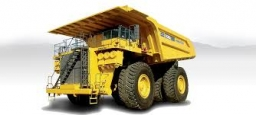# Transported 23301

The material, which weighed 18.2 tons, was transported by three cars. The second drove 20% more than the first and the third 20% more than the second. How many tons did one car transport?

a =  5
b =  6
c =  7.2

### Step-by-step explanation:

a+b+c = 18.2
b = 0.20a+a
c = 0.20b+b

a+b+c = 18.2
b = 0.20·a+a
c = 0.20·b+b

a+b+c = 18.2
1.2a-b = 0
1.2b-c = 0

Pivot: Row 1 ↔ Row 2
1.2a-b = 0
a+b+c = 18.2
1.2b-c = 0

Row 2 - 1/1.2 · Row 1 → Row 2
1.2a-b = 0
1.833b+c = 18.2
1.2b-c = 0

Row 3 - 1.2/1.83333333 · Row 2 → Row 3
1.2a-b = 0
1.833b+c = 18.2
-1.655c = -11.913

c = -11.91272727/-1.65454545 = 7.2
b = 18.2-c/1.83333333 = 18.2-7.2/1.83333333 = 6
a = 0+b/1.2 = 0+6/1.2 = 5

a = 5
b = 6
c = 36/5 = 7.2

Our linear equations calculator calculates it.Did you find an error or inaccuracy? Feel free to write us. Thank you!

Tips for related online calculators
Do you have a system of equations and looking for calculator system of linear equations?